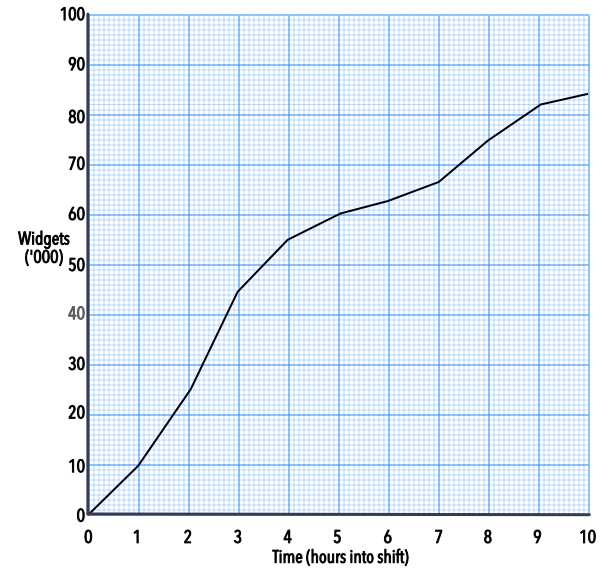Gradients of Graphs as Rates of Change

# Gradients of Graphs as Rates of Change

GCSE(F), GCSE(H),

The gradient on a graph gives the rate of change of one measure against another.

The steeper the gradient, the higher the value of the rate of change. If a gradient has a negative value, then the rate of change is negative.

For example, a graph shows the cumulative number of widgets produced against the time in a shift. The gradient between any two times gives the rate of production for that time.The average rate of production for the shift can be obtained by drawing a line from the origin to the highest point on the graph.

## Examples

1. Using the graph, above, what is the rate of production between the 2nd and 3rd hour?

Answer: 20 000 widgets / hour

Note that the vertical scale is in thousands of widgets.

The gradient of the graph is given as frac(text(up))(text(along))

= frac(45000 - 25000)(3 - 2)

= 20 000 widgets / hour

2. Using the graph, what is the average production for the first 4 hours?

Answer: 13750 widgets / hour

The gradient of the graph is given as frac(text(up))(text(along))

= frac(55000 - 0)(4)

= 13750 widgets / hour